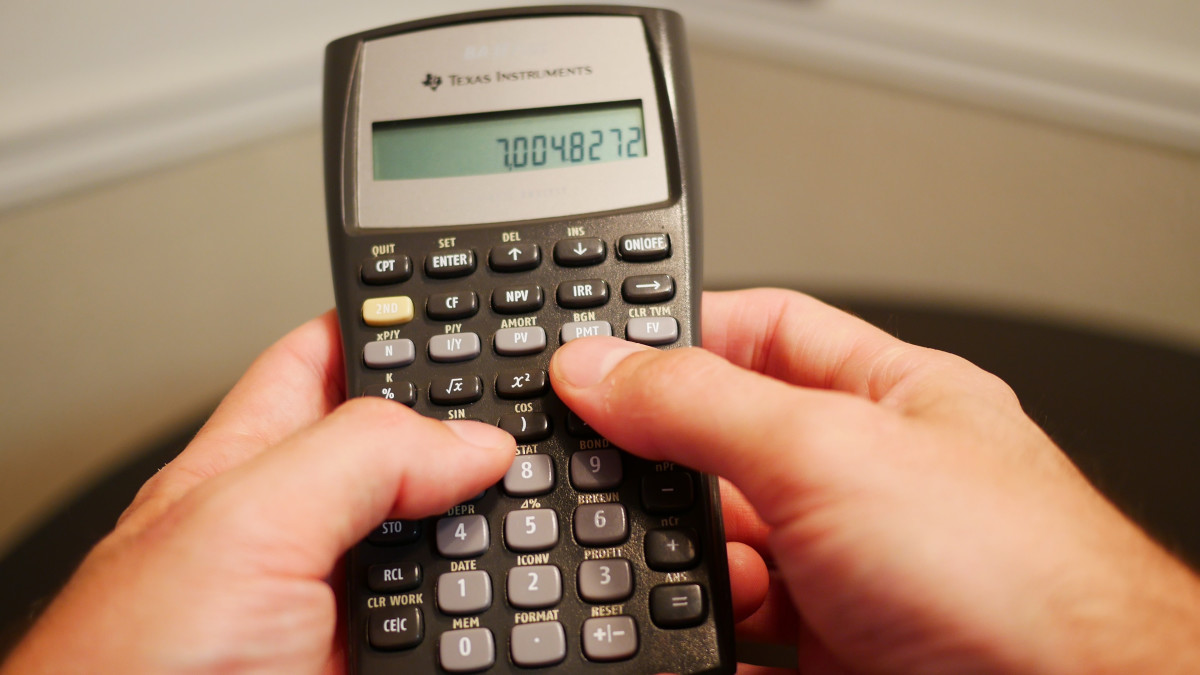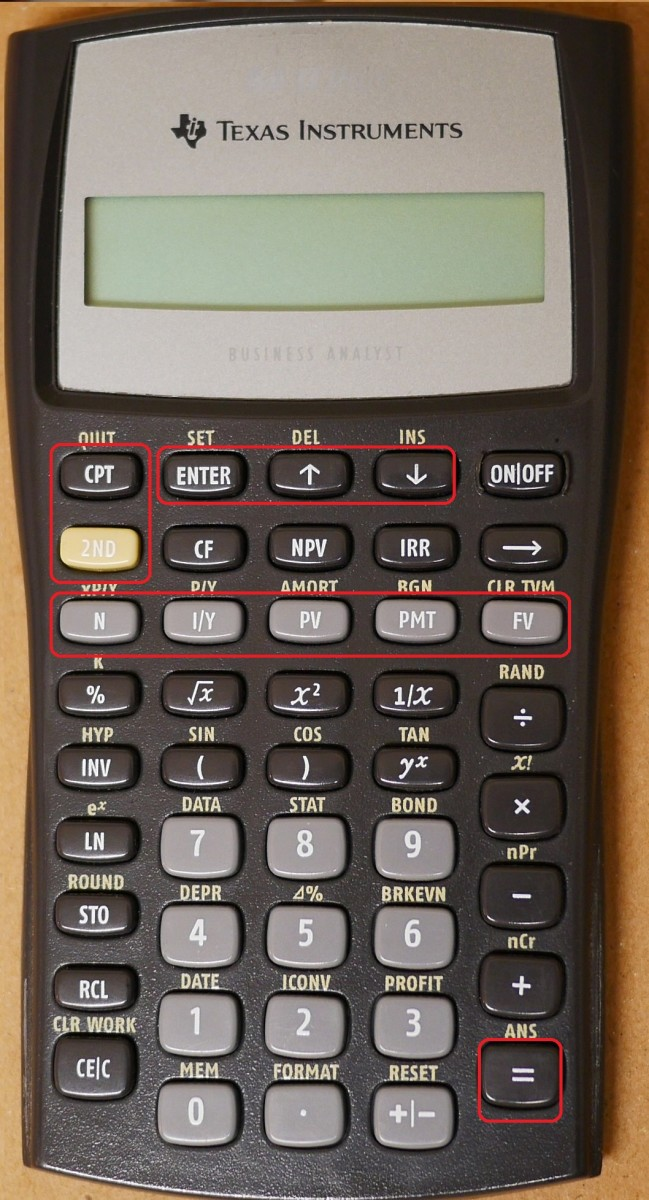# How to Answer Amortization Problems With a TI BAII+ Calculator

Joshua earned an MBA from USF and he writes mostly about software and technology.

## TI BA II Plus CalculatorThe TI BA II Plus has multiple features for business use. For instance, the ability to compute TVM calculations, create amortization schedules, complete a Cash-flow analysis, compute Net Present Value, compute Internal Rate of Return, and more.Joshua Crowder

## What Is Amortization?

Amortization occurs during periodic payments for a debt where the payment must be paid on a certain date. Included in these payments are interest and a portion of principal. Good examples of where amortization occurs are with transactions such as mortgages and car loans.

Solving amortization problems can be easy if you have all of the correct and necessary data. Normally you have to organize your data into a table. This can become very time-consuming for a problem that is amortized over the course of 10 years! As long as you have enough information to calculate the payment, you will be able to reference all relevant data with the TI BAII+ calculator.

In order to get similar answers when you follow along, make sure that your calculator is set up for annual interest per year. If you need to amortize using an HP 10bII+ calculator, click here.

## Button Use for Time Value of MoneyThe time value of money keys on this calculator is on the third row down. With these keys, one can compute present values, future values, years to accrue, payments, and interest. Created by Joshua Crowder

## Sample Problem

Let’s say you borrow \$100,000 and make annual payments for three years. If the interest rate is 7%, how much interest do you pay in year two?

The first thing we want to do is clear the time value of money buttons on the calculator. Press the 2ND button, then press the CLR TVM (FV) button.

1. Type 3, then press the "N" button (There are three payment periods)
2. Type 7, then press the "I/YR" button (Do not input the symbol %)
3. Type 100,000, then press the "PV" button (This is positive because cash is received)
4. Type 0, then press the "FV" button (If you cleared your calculator when you started you can skip this step)

After pressing the N, I/YR, PV, and FV buttons, the inputted data will be saved in the calculator.

## Display the Data

To display the fixed payment, press the CPT button, then press the "PMT" button. Your calculator should display a cash outflow of -38,105.17. This is the annual payment that you would have to pay to borrow this money. Now that we know the fixed payment, let's look at the other details. Press the 2ND button, then press the "AMORT" button. The calculator will display "P1 =" and has a number to the right of the screen. To display data for period 1, press 1, then press the enter button. Next, you must press the down arrow button and set P2 to 1 as well. Press the down button again and you will start to cycle through period 1 data. This data is displayed for you below:

Principle -31,105.17

Interest -7,000.00

Balance 68,894.83

Now that you have reviewed period 1 data, let's see what period 2 data holds. Press the down button to get to P1 and P2 and set them both to 2. Now you can cycle through period 2 data with the down button. This data is displayed for you below:

Scroll to Continue

Principle -33.282.53

Interest -4,822.64

Balance 35,612.31

To answer the problem above, interest paid in year two would be \$4,822.64. Remember that your answer should remain a positive number even though your calculator displays the number as a negative cash flow. To view period 3 data, set P1 and P2 to 3.

Below is an amortization table/schedule created in MS Excel. This table took me about eight minutes to create in Excel. With the calculator, I was able to complete the table in about three minutes.

## Monthly Payment Example

Annual payments were made in our example above. Let's try the same problem but change annual payments to monthly payments. In this example, you must find the number of periods and the periodic rate. This can be done by completing the calculation right before entering data to the "N" or "I/YR" buttons.

If the problem states that monthly payments would be made, then we would have to multiply 3 times 12 to give us 36 total payments. So immediately after we make this calculation, we can press the "N" button. Now that we are making 36 payments over the course of 3 years, our payment should be significantly smaller. Next, we have to figure out the periodic interest rate. This can be found by dividing the interest by the number of periods per year. When entering interest, type 7 / 12 = and then press the "I/YR" button. See below for data entry instructions.

1. Type 3 x 12 =, then press the "N" button
2. Type 7 / 12 =, then press the "I/YR" button
3. Type 100,000, then press the "PV" button
4. Type 0, then press the "FV" button

When you press the "CPT" button, then the "PMT" button to retrieve the value of the payment, the result should be -3,087.71 and your interest payment in period 2 would be \$568.72.

## Quarterly Payment Example

If the problem stated that quarterly payments would be made, then we would have to multiply 3 times 4 to give us 12 total payments. The periodic interest rate can be found by dividing the interest by the number of periods per year. When entering interest, type 7 / 4 = and then press the "I/YR" button. See below for data entry instructions.

1. Type 3 x 4 =, then press the "N" button

2. Type 7 / 4 =, then press the "I/YR" button

3. Type 100,000, then press the "PV" button

4. Type 0, then press the "FV" button

When you press the "CPT" button, then the "PMT" button to retrieve the value of the payment, the result should be -9,311.38 and your interest payment in period 2 would be \$1,617.68.

Cornell Law School. (n.d.). Amortization. Retrieved January 14, 2020, from https://www.law.cornell.edu/wex/amortization.

This content is accurate and true to the best of the author’s knowledge and is not meant to substitute for formal and individualized advice from a qualified professional.